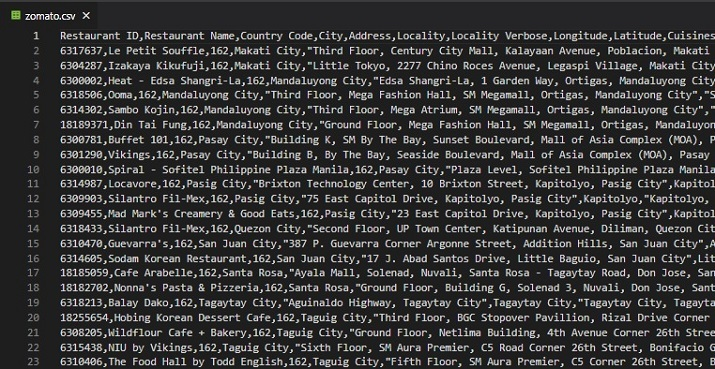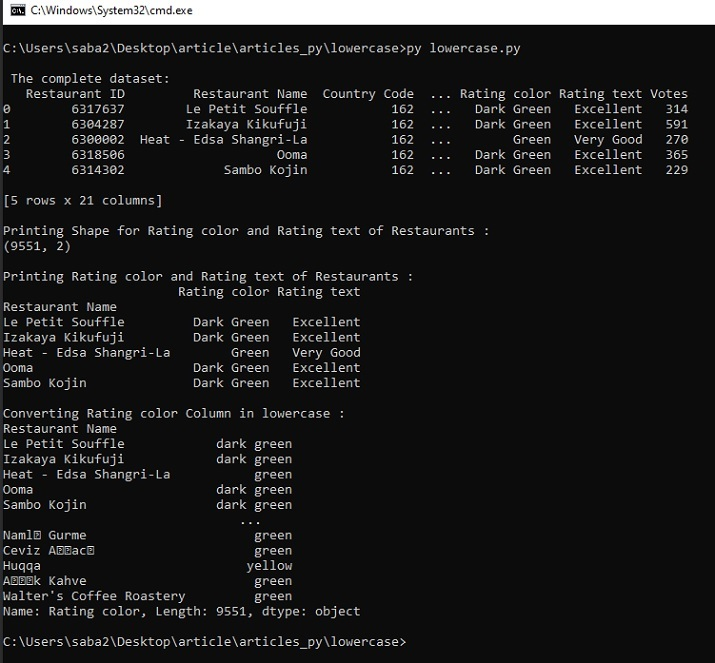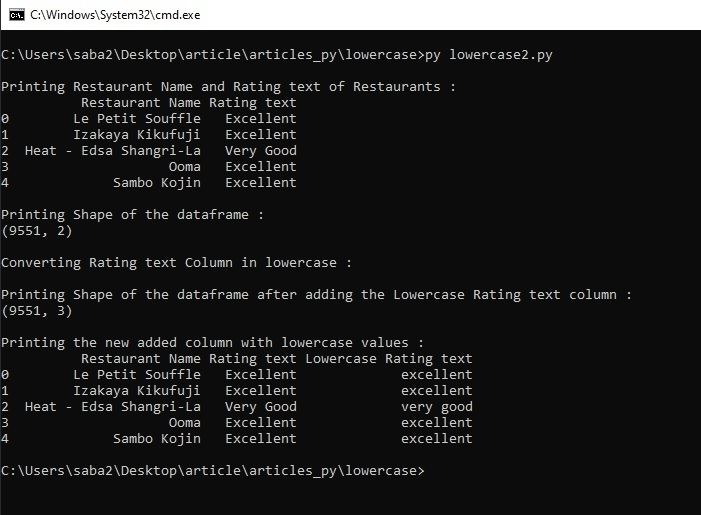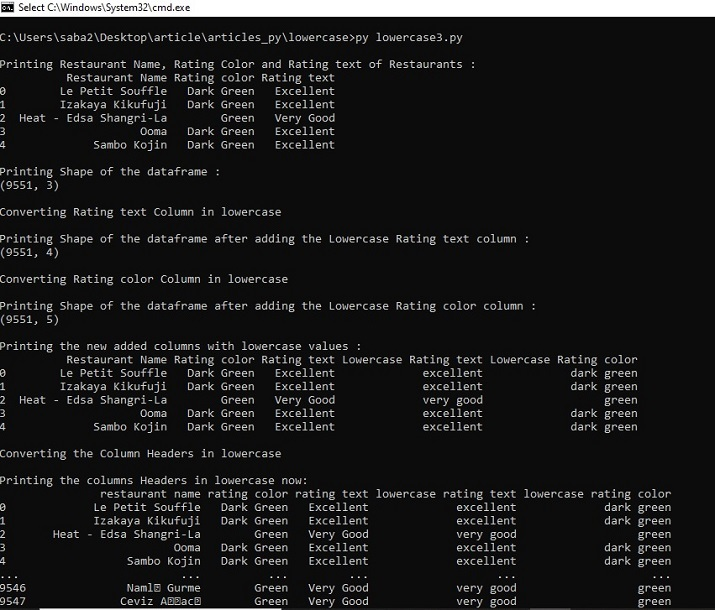# How to lowercase the column names in Pandas dataframe?

In this article, the user will understand how to lowercase the column names in Pandas dataframe. Using three different examples, the ways are given for converting the dataframe columns in lowercase. For these examples, a Zomato dataset given on Kaggle is used. The kaggle dataset was available in CSV (Comma Separated Values) format so it was first downloaded and then it was converted into a dataframe by using pandas.

In the first example, the python program uses a str.lower() function for the lowercase conversion of the column vales. In the second example, the map(str.lower) function is used for converting the dataframe columns into lowercase. The third example has two parts. First, the method is given to change the column contents in lowercase by using apply(lambda x: x.lower()) function and then the method is given to convert the column headers in lowercase by using map(str.lower, dataframe.columns).

## Saving the data file / csv file required for data analysis

For these examples, we will use the data available on Kaggle. Login to Kaggle and the download the csv file from this linkhttps://www.kaggle.com/datasets/shrutimehta/zomato-restaurants-data

The dataset is available as a CSV file.

### The Zomato.CSV file usedFig: This csv file contains 9551 rows and 21 columns.

## Example 1: Using str.lower() function on dataframe columns to convert the content to lowercase

Design Steps and Coding

• Step 1 − First import pandas. Now read the zomato.csv file as the dataset given here will be used for loading it into the dataframe.

• Step 2 − Make a dataframe dff1 and use the read_table function from pandas for reading the CSV file. Now create another dataframe dff2 similarly, but using the restaurant name as the index column.

• Step 3 − Use the delimiter=',' and the path to the zomato.csv for these. Print some rows and columns from this dataframe by using the head function.

• Step 4 − Select some columns from dff2 that need to be converted into lowercase. This new dataframe is dff3. Apply str.lower()) on one of the columns of dff3 and convert it to lowercase.

• Step 5 − Run the program and check the result.

### Write the following code in the python file

import pandas as pdd
print("\n The complete dataset: ")

#print(" \nThe complete dataset with specified index : ")
dff3=dff2[["Rating color", "Rating text"]]
print("\nPrinting Shape for Rating color and Rating text of Restaurants : ")
print(dff3.shape)
print("\nPrinting Rating color and Rating text of Restaurants : ")

print("\nConverting Rating color Column in lowercase : ")
print(dff3['Rating color'].str.lower())


## Output

Run the python file in the Command windowFig 1: Showing the results using cmd window.

## Example 2: Using map(str.lower) function on dataframe columns to convert the content to lowercase

Design Steps and Coding

• Step 1 − First import pandas. Now read the zomato.csv file as the dataset given here will be used for loading it into the dataframe.

• Step 2 − Make a dataframe dff2 and use the read_csv function from pandas for reading the CSV file. Just choose two columns, 'Restaurant Name', and 'Rating text' by using usecols.

• Step 3 − Use the delimiter=',' and the path to the zomato.csv for these. Print some rows and columns from this dataframe by using the head function.

• Step 4 − Apply the map(str.lower) on one of the columns of dff2 and convert it to lowercase.

• Step 5 − Run the program and check the result.

### Write the following code in the python file

import pandas as pdd

dff2 = pdd.read_csv('C:/Users/saba2/Desktop/article/articles_py/tsv/zomato.csv', sep=',',usecols=['Restaurant Name', 'Rating text'])

print("\nPrinting Restaurant Name and Rating text of Restaurants : ")

print("\nPrinting Shape of the dataframe : ")
print(dff2.shape)

print("\nConverting Rating text Column in lowercase : ")
dff2["Lowercase Rating text"]= dff2['Rating text'].map(str.lower)

print("\nPrinting Shape of the dataframe after adding the Lowercase Rating text column : ")
print(dff2.shape)
print("\nPrinting the new added column with lowercase values : ")


## Output

Run the python file in the Command windowFig 2: Showing the results using cmd window.

## Example 3: Changing the column headers and content in lowercase

Design Steps and Coding

• Step 1 − First import pandas. Now read the zomato.csv file as the dataset given here will be used for loading it into the dataframe.

• Step 2 − Creation of a dataframe named dff2 and utilize the read_csv function from pandas for reading the CSV file. Select three columns, 'Restaurant Name', 'Rating text', and ‘Rating color’ by using usecols.

• Step 3 − Utilize the delimiter=',' and the path to the zomato.csv for these. Print some rows and columns from this dataframe by using the head function.

• Step 4 − Use the apply(lambda x: x.lower()) on the 'Rating text' of dff2 and convert it to lowercase. Add this lowercase column to the dataframe.

• Step 5 − Use Step 4 on the “Rating color” column now.

• Step 6 − Apply the map(str.lower, dataframe.columns) function on dataframe columns to convert the column headers to lowercase.

• Step 7 − Run the program and check the result.

### Write the following code in the python file

import pandas as pdd

dff2 = pdd.read_csv('C:/Users/saba2/Desktop/article/articles_py/tsv/zomato.csv', sep=',',usecols=['Restaurant Name', 'Rating color', 'Rating text'])

print("\nPrinting Restaurant Name, Rating Color and Rating text of Restaurants : ")

print("\nPrinting Shape of the dataframe : ")
print(dff2.shape)

print("\nConverting Rating text Column in lowercase")
dff2["Lowercase Rating text"]= dff2['Rating text'].apply(lambda x: x.lower())
print("\nPrinting Shape of the dataframe after adding the Lowercase Rating text column : ")
print(dff2.shape)

print("\nConverting Rating color Column in lowercase")
dff2["Lowercase Rating color"]= dff2['Rating color'].apply(lambda x: x.lower())
print("\nPrinting Shape of the dataframe after adding the Lowercase Rating color column : ")
print(dff2.shape)

print("\nPrinting the new added columns with lowercase values : ")

print("\nConverting the Column Headers in lowercase")
dff2.columns = map(str.lower, dff2.columns)
print("\nPrinting the columns Headers in lowercase now: ")
print(dff2)


## Output

Run the Python file in the Command window.Fig 3: Showing the results using cmd window

## Conclusion

In this Python and Pandas article, we use three different examples, to demonstrate the ways how to convert the values of a dataframe column into lowercase. For these different functions are used in all three examples. In the third example, the method is also given to change the column headers in the lowercase.

Updated on: 11-May-2023

1K+ Views